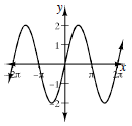### Home > A2C > Chapter 9 > Lesson 9.3.3 > Problem9-176

9-176.

Graph two cycles of each function.

1. $y = - 2 \operatorname { cos } ( x + \frac { \pi } { 2 } )$

What is the amplitude? Where is the locator point?1. $y = \operatorname { sin } ( x - \frac { \pi } { 2 } )$

See part (a).